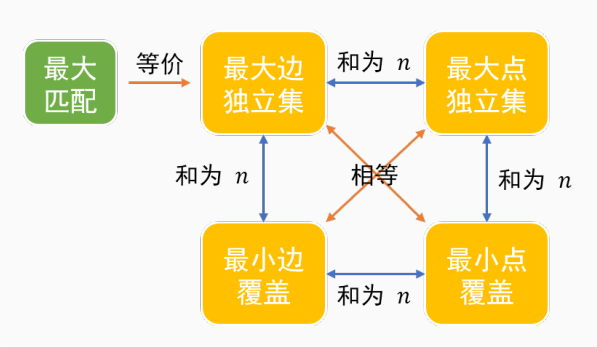# 二分图

Bipartite graph, 又称二部图

• 尝试用两种颜色标记节点，如果有一个点的染色产生了冲突，那么这个图就不是二分图

• 染色结束之后，$$A$$, $$B$$集合中的点分别为黑色点集以及白色点集

## 二分图的最大匹配

### 匈牙利算法（增广路算法）

#### 算法流程

1. $$S = \empty$$ ，即所有边都是非匹配边
2. 寻找一条足够长的增广路，把路径上的所有状态取反：匹配边变为非匹配边，非匹配边变为匹配边
3. 重复上述步骤，直到图中不存在增广路

1. 遍历每一个结点

2. 尝试当前结点 $$s$$ 和一个点 $$t$$ 匹配，如果 $$t$$ 以及被匹配，尝试更换 $$t$$ 的匹配。注意：在最外层遍历时，每一次每一个点只能被匹配一次，总共最多会匹配 $$O(n)$$

3. 重复上述步骤，使得所有点都被遍历到

int match[N], vis[N], vt = 0;
bool dfs(int x) {
// 采用链式前向星的写法
for (int y, i = head[x]; i; i = edge[i].next)
// 在每一次遍历中，一个点只会访问一次
if (!vis[(y = edge[i].to)]) {
vis[y] = vt;
if (!match[y] || dfs(match[y]) {
match[y] = x; return true;
}
}
return false;
}

int main() {
// ....

int matchCount = 0;
// vt: visit time, 用于避免memset的使用
for (vt = 1; vt <= n; ++vt) {
if (dfs(vt)) ++matchCount;
}

// ....
return 0;
}


## 二分图的覆盖于独立集

• 最小边覆盖：你可以认为是用最少的边覆盖所有的点。选择尽量少的边，使得任意一个点相连的边中至少有一条边被选中

• 最小点覆盖： 同理，用最少的点覆盖所有的边。选择尽量少的点，使得任意一条边的两个端点至少有一个被选中

• 最大点独立集：在不重复覆盖一条边的情况下，选择最多的点。选择尽量多的边，使得任意一条边的两端至多有一个点被选

• 最大边独立集：在不重复覆盖一个点的情况下选择最多的边。这不就是最大二分匹配？

• DAG的最小路径点覆盖：感觉没啥用。用最少的路径，不重复的覆盖所有的点

### 关系

graph LR; A[A最大匹配] B[B最大边独立集] C[C最大点独立集] D[D最小点覆盖] E[E最小边覆盖] A ----|等价| B B ----|和为N| E B ----|相等| D B ----|和为N| C C ----|和为N| D C ----|相等| E D ----|和为N| E## 参考题目

• CH6801
• CH6901
• CH6902
• POJ1325
• POJ2226
• ZJOI2007 矩阵游戏
• NOIP2010 关押罪犯
posted @ 2023-01-13 21:50  jeefy  阅读(54)  评论(0编辑  收藏  举报